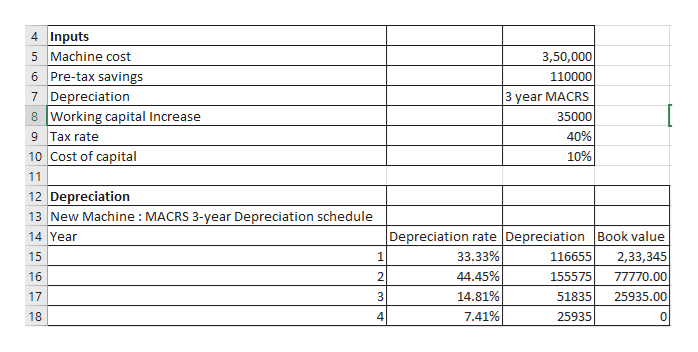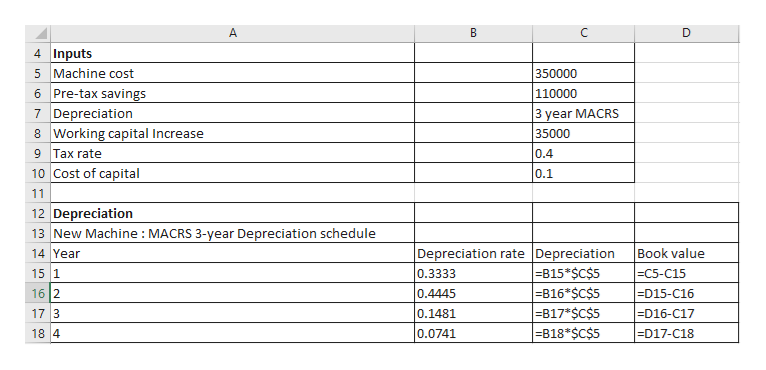Question

New-Project Analysis

Madison Manufacturing is considering a new machine that costs \$350,000 and would reduce pre-tax manufacturing costs by \$110,000 annually. Madison would use the 3-year MACRS method to depreciate the machine, and management thinks the machine would have a value of \$33,000 at the end of its 5-year operating life. The applicable depreciation rates are 33.33%, 44.45%, 14.81%, and 7.41%. Working capital would increase by \$35,000 initially, but it would be recovered at the end of the project's 5-year life. Madison's marginal tax rate is 40%, and a 10% cost of capital is appropriate for the project.

1. Calculate the project's NPV. Round your answer to the nearest dollar.

Calculate the project's IRR. Round your answer to two decimal places.
%
Calculate the project's MIRR. Round your answer to two decimal places.
%
Calculate the project's payback. Round your answer to two decimal places.

2. Assume management is unsure about the \$110,000 cost savings - this figure could deviate by as much as plus or minus 20%. Calculate the NPV if cost savings value deviate by plus 20%. Round your answer to the nearest dollar.

Calculate the NPV if cost savings value deviate by minus 20%. Round your answer to the nearest dollar.
\$

3. Suppose the CFO wants you to do a scenario analysis with different values for the cost savings, the machine's salvage value, and the working capital (WC) requirement. She asks you to use the following probabilities and values in the scenario analysis:

 Scenario Probability CostSavings SalvageValue WC Worst case 0.35 \$  88,000 \$28,000 \$40,000 Base case 0.35 110,000 33,000 35,000 Best case 0.30 132,000 38,000 30,000

Calculate the project's expected NPV. Round your answer to the nearest dollar.

Calculate the project's standard deviation. Round your answer to the nearest dollar.

Calculate the project's coefficient of variation. Round your answer to two decimal places.

Step 1

As per the guidelines, we can answer the first question as it has multiple sub-parts. Request you to repost the question mentioning which parts to answer for our experts to answer them.

Step 2

Project analysis is important to understand if the project would be of benefit to the organization or not. This is the reason why Net Present Value (NPV), Internal rate of return (IRR), Modified Internal rate of return (MIRR) and Payback period is used.

Operating cash flow of the project is first determined followed by calculating the NPV, IRR, MIRR and payback period of a projecthelp_outlineImage Transcriptionclose4 Inputs 5 Machine cost 6 Pre-tax savings 7 Depreciation 8 Working capital Increase 3,50,000 110000 3 year MACRS 35000 40% 9 Tax rate 10 Cost of capital 10% 11 12 Depreciation 13 New Machine MACRS 3-year Depreciation schedule Depreciation rate Depreciation Book value 14 Year 33.33% 116655 2,33,345 15 1 44.45% 16 2 155575 77770.00 25935.00 14.81% 17 3 51835 7.41% 18. 25935 0 n fullscreen
Step 3

Calculatio...help_outlineImage TranscriptioncloseA D 4 Inputs 5 Machine cost 6 Pre-tax savings 7 Depreciation 8 Working capital Increase 9 Tax rate 10 Cost of capital 350000 110000 3 year MACRS 35000 0.4 0.1 11 12 Depreciation 13 New Machine: MACRS 3-year Depreciation schedule Depreciation rate Depreciation -B15*\$C\$5 -B16* \$C\$5 -B17*\$C\$5 -B18*\$C\$5 14 Year Book value 15 1 0.3333 |ЕC5-C15 16 2 D15-C16 0.4445 17 3 0.1481 D16-C17 18 4 0.0741 D17-C18 fullscreen

Want to see the full answer?

See Solution

Want to see this answer and more?

Our solutions are written by experts, many with advanced degrees, and available 24/7

See Solution
Tagged in